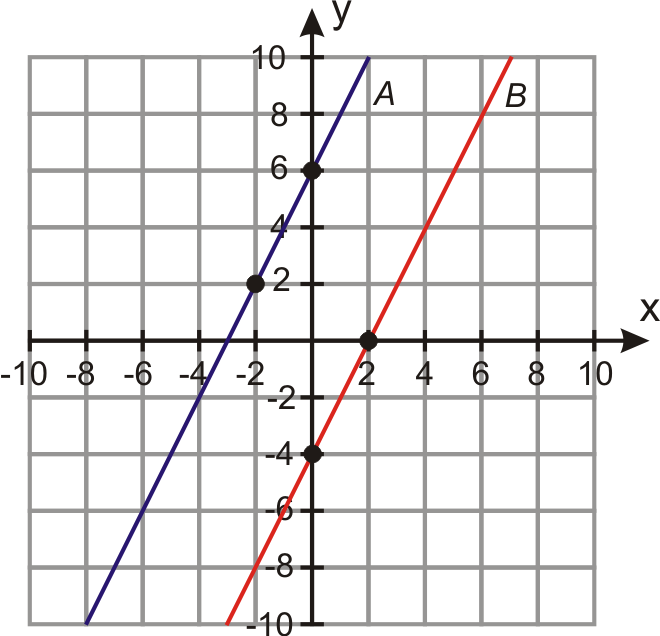Determining if two lines are parallel (Graphically and algebraically)

Complete the form below to get homework credit
This is a required question
This is a required question
This is a required question
This is a required questionThis is a required question
This is a required question
This is a required question
This is a required question
This is a required question